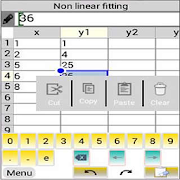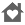# Curve Fitting & Matrix SolverEveryone
2This Mathematical program contains the following powerful tools:
- Robust Non Linear Fitting (Regression) Tool
- Interpolation Calculator
- Matrix Calculator
- Periodic Table

The recent version contains many features and with user interface improved.

Curve fitting tool performs statistical regression analysis to estimate the values of parameters for linear, multivariate, polynomial, and nonlinear functions. The regression analysis determines the values of the parameters that cause the function to best fit the observed data that you provide.

Non Linear Fitting Tool (NLFT) is the most powerful tool to find the values of all coefficients of any non linear function. The observational data are modeled by a function which is a nonlinear combination of the model parameters and depends on one or more independent variables(the number of variables or parameters are not limited). The data are fitted by a robust algorithm. The tool is unique in its kind available in android phones and tablets.

1-D cubic spline interpolation of x/y-values at xi. interpolates to find yi, the values of the underlying function y at the points in the array xi.

Matrix calculator: determinant calculation, matrix inverse, matrix multiplication and systems of linear equations.

The tools have a very smart user interface system allows easy editing and viewing data.

Key Features:

Linear & polynomial fitting
• Find the best-fit line or polynomial curve to fit your input data
• Multiplelinear regression up to 10 independent variables
• Flexible data entry
• Opening and saving data

Nonlinear fitting (Nonlinear Regression)
• User defined regression model (input function) can have many fitting parameters, or variables, and you can use whatever variable names you want to use in your input function.
• The tool implements the Nelder-Mead simplex (direct search) algorithm, to minimize a non linear function of several variables.
• Flexible data entry
• Opening and saving data

Matrix solver
• Solving a linear equation system of up to 20 unknowns
• Calculate the determinant, inverse of the given input of a matrix
• Matrix multiplication
• Flexible data entry
• Opening and saving data

Supported languages included:
1. English
2. French
3. German
4. Italian
5. Spanish
6. Portuguese

For more details please visit the website: http://www.mobile-sciences.com
Collapse

## Reviews

Review Policy
2.5
2 total
5
4
3
2
1

Eligible for Family LibraryEligible if bought after 7/2/2016. Learn More
Updated
November 16, 2018
Size
12M
Installs
50+
Current Version
81.0
Requires Android
4.1 and up
Content Rating
Everyone
Permissions
Offered By
mobile-sciences
Developer
Rue AI no: 3 Bouakel 3 Batna 05000 Italie